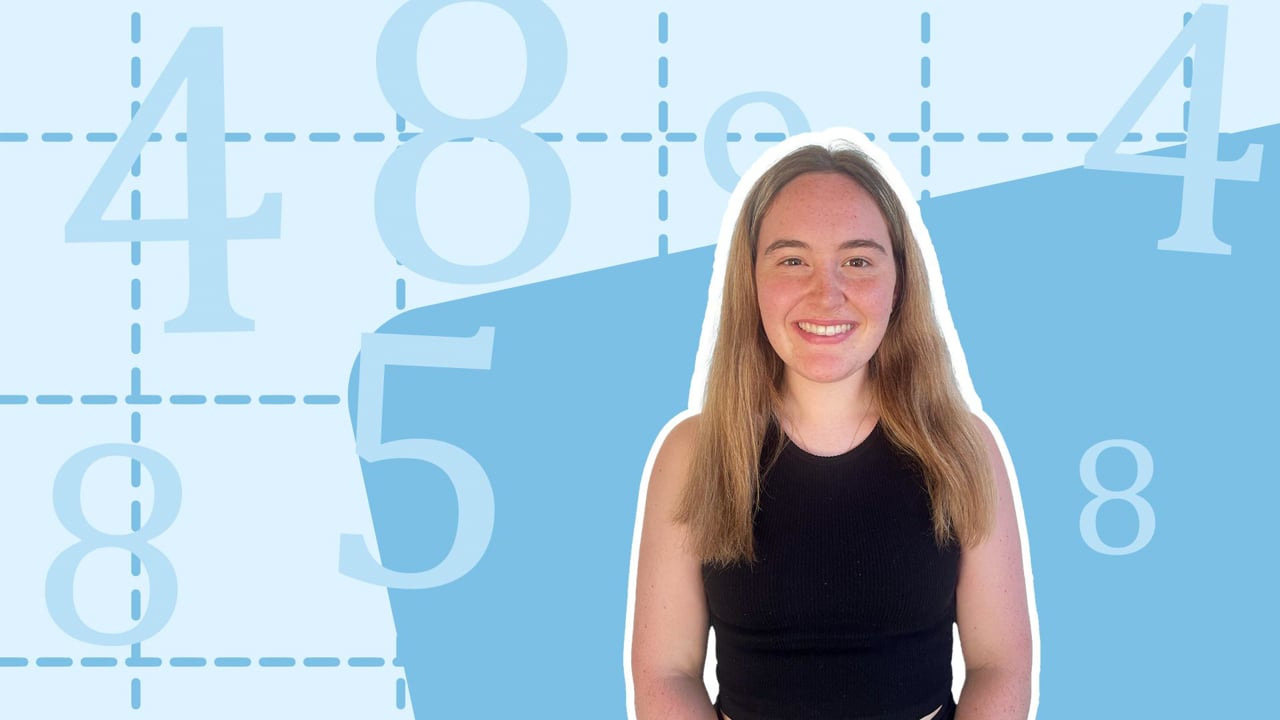Chapter OverviewMaths

Number and place value

Multiplication and division

Fractions

Measurement

Geometry - properties of shapes

Geometry - position and direction

Statistics

Ratio and proportion

Algebra

Maths

# Calculating percentages of amounts0%

Summary

# Calculating percentages of amounts

## ​​In a nutshell

It is often useful to calculate percentages of amounts when solving problems. Some are simple and some require a little more work.

## Finding percentages of amounts

There are two methods to calculating percentages of amounts: by using simple percentages that you already know, or by converting the percentage to a fraction and multiplying.

### Method 1: Using simple percentages

You can always split up a percentage into smaller simple ones. Below are some simple percentages that you need to remember how to calculate, as well as their equivalent fraction pairs.

 PERCENTAGE FRACTION HOW TO CALCULATE $50 \%$​​ ​$\frac{1}{2}$​​ Divide by two ​$25\%$​​ ​$\frac{1}{4}$​​ Divide by four ​$10\%$​​ ​$\frac{1}{10}$​​ Divide by ten ​$5\%$​​ ​$\frac{1}{20}$​​ Divide by ten and then two ​$1\%$​​ ​$\frac{1}{100}$​​ Divide by one hundred

##### Example 1

In a sale, everything is $17\%$ off. What saving would be made on $£200$?​

Split $17\%$ into simple percentages.

$17\%=10\%+5\%+1\%+1\%$

Work out each of the simple percentages.

$10\%$ of $200 = 200\div10=20$

$5\%$ of $200= 10\% \div 2=20\div2=10$

$1\%$ of $200= 200\div100=2$

​​​

$20+10+2+2=\underline{£32}$

### Method 2: Turn into a fraction and multiply

The second method is to simply turn the percentage into a fraction and multiply by the amount. Remember that to convert from a percentage to a fraction you take the percentage as a fraction out of one hundred.

##### Example 2

Calculate $29\%$ of $£3300$.

Write $29\%$ as a fraction out of one hundred.

$\frac{29}{100}$​​

Multiply by $£3300$.

\begin{aligned}\frac{29}{100}\times£3300=£3300 \div 100 \times 29\\=£33 \times 29 = \underline{£957} \end{aligned}

## Want to find out more? Check out these other lessons!

Mean, median, mode and range

FAQs

• Question: How do I work out a percentage using fractions?

Answer: Turn the percentage into a fraction and multiply by the amount. To convert from a percentage to a fraction you take the percentage as a fraction out of one hundred.

• Question: How do I work out 1% of a number?

Answer: To work out 1% of a number, divide by one hundred.

• Question: How do I work out 10% of a number?

Answer: To work out 10% of a number, divide by ten.

• Question: How do I work out 50% of a number?

Answer: To work out 50% of a number, divide by two.

• Question: How do I find a percentage of an amount?

Answer: There are two methods to calculating percentages of amounts: by using simple percentages that you already know, or by converting the percentage to a fraction and multiplying.

Theory

Exercises